Articulo

•Citado por SciELO
•Accesos

•Citado por Google
•Similares en SciELO
•Similares en Google

versión impresa ISSN 0012-7353

Dyna rev.fac.nac.minas vol.86 no.208 Medellín ene./mar. 2019

http://dx.doi.org/10.15446/dyna.v86n208.67454

Artículos

Numerical comparisons of sandwich viscoelastic beam models

Comparaciones numéricas de modelos de vigas sándwich con núcleo viscoelástico

Waldir Felippea  , Flávio Barbosab  , Carlos Maglutac  , Ney Roitmanc  , Flávia Borgesd

a Facultad de Ingenería Civil, Universidad de Barra Mansa, Barra Mansa, Brasil. waldir.felippe@gmail.com

b Facultad de Ingeniería Civil, Universidad de Juiz de Fora, Juiz de Fora, Brasil. flavio.barbosa@ufjf.br

c Programa de Ingeniería Civil - COPPE, Universidad Federal del Rio de Janeiro, Rio de Janeiro, Brasil. magluta@coc.ufrj.br, roitman@coc.ufrj.br

d Facultad de Ingeniería Civil, Universidad Veiga de Almeida, Rio de Janeiro, Brasil. fclborges@hotmail.com

Abstract

Composite structures with elastic layers and viscoelastic core have been used as a passive damping treatment applied to reduce vibration amplitudes. In the design phase of this type of damping technique, many aspects ranging from computer modeling to laboratory tests should be considered. Due to the frequency dependency of mechanical properties on these materials, time domain based models for viscoelastic materials are not as numerous as frequency domain based methods. Usually, time domain methods introduce extra dissipation coordinates or internal variables as Golla-Hughes-McTavish (GHM) method and also Anelastic Displacement Field (ADF) method. In this paper, these methods are evaluated by comparing their results to the ones obtained by means of theoretical analysis. Processing time are also evaluated highlighting advantages and disadvantages of these methodologies. Finally, these two-time domain methods are applied to a real structure, pointing out the facilities and difficulties to simulate an actual situation.

Key words: viscoelastic material; sandwich model; vibration control

Resumen

Estructuras compuestas con capas elásticas y núcleo viscoelástico han sido usadas como tratamiento amortiguador pasivo para reducir las amplitudes de vibraciones. En el proyecto de este tipo de técnica de amortiguación, muchos aspectos deben ser considerados, desde este modelaje computacional hasta ensayos en laboratorio. En este artículo, un abordaje basado en los modelos Golla-Hughes-McTavish (GHM) y Anelastic Displacement Field (ADF) son presentados y aplicados al modelaje de materiales viscoelásticos. Con el fin de evaluar los resultados numéricos se realizó un programa experimental. En este sentido, a partir de las propiedades dinámicas de un material viscoelástico, son evaluados el comportamiento de estructuras sándwich a través de modelos numéricos, enseñándonos las ventajas y desventajas de estas metodologías.

Palabras-clave: material viscoelástico; modelo sándwich; control de vibraciones

1. Intoduction

Structural vibrations are undesirable not only for the discomfort caused to users, but also for the fatigue process, which is accelerated by dynamic oscillations. These effects may be detected specifically in structures with low stiffness and low natural frequencies, leading to large displacement amplitudes.

Aiming the reduction of structural vibrations, several techniques were developed to increase structural damping. Among these techniques, the passive control with viscoelastic materials has shown reasonable efficiency. In order to effectively reduce structural vibrations using this technique, it is important to understand the dynamic behaviors of the structure and of the viscoelastic material (VEM).

Due to the mechanical properties frequency dependence on VEM, time domain based models are not so numerous as frequency domain methods. In spite of that, because of the facilities that time domain methods may directly provide, such as the maximum displacement range in a structural model analysis, many researchers have been developing numerical methods to simulate the VEM dynamical response in the time domain. The most successful models are the ones that introduce extra dissipation coordinates or internal variables in a Finite Element, due to its simplicity and capability to virtually model any complex geometry. This kind of methodology has been applied in several situations such as the ones presented by Friswell et. al , Roy et. al , Wang et. al  and Wang et. al . Among the dissipation coordinates based methods it is possible to observe that Golla-Hughes-McTavish (GHM) method [5,6] and Anelastic Displacement Field (ADF) method [7-11] are frequently chosen in order to simulate the dynamic response of VEM.

Within this context, this paper will discuss the computational modeling of VEM and their use for reducing vibrations in structures, working as a passive control mechanism in sandwich layers. Computational viscoelastic sandwich models, based on GHM and ADF methods, are analyzed and their results are compared with theirs theoretical counterparts. Finally, an actual structure is used to evaluate the facilities and difficulties of each applied technique.

2. Viscoelastic materials modelling

2.1. The GHM model

The stress-strain relation for a single degree of freedom system on Laplace’s domain, as mentioned by reference , may be written as:

where s is the Laplace operator, σ

σ(s) and are, respectively, the stress and strain on Laplace’s domain, E 0 is the elastic fraction of complex modulus and h(s) is the relaxation function.

Function h(s) can be written using Biot’s  series, with two terms as:

The GHM model is developed starting from the equation of motion in the Laplace domain:

where, M L , K L and f L are respectively the mass, stiffness and external loading in the Laplace domain, being:

and K v is the rigidity associated with geometrical characteristics of the model.

The GHM model defines the equation of motion in the time domain as:

where:

i is the auxiliary variable introduced into the problem, called dissipation variable, and .

Generalizing eq. (5) for n degrees of freedom, then this equation may be written as in eq. (6):

and where:

being Λ a diagonal matrix consisting of the non-zero eigen-values of the stiffness matrix normalized with respect to the elastic modulus, E; Tthe matrix of vectors corresponding to the non-zero eigen-values of the matrix 1/E K elastic , where K elastic the elastic Finite Element stiffness matrix is; R=T Λ 1/2 and

As shown in eq. (2) and (6) the number of dissipative degrees of freedom associated with viscoelastic elements depends on the number of terms used in relaxation function and the number of rigid body motions . It should be noted that the greater the number of terms used to write the relaxation function the more accurate the modelling.

Using eq. (6) and (7), it is possible to determine stiffness, mass and damping matrices, for any kind of Finite Element.

2.2. The ADF model

Lesieutre  establishes that the displacement field can be written as:

where q e(t) is the elastic displacement field and q a j (t) is the j-th anelastic displacement field are then the strain field is defined as:

The ADF model defines the equation of motion in the time domain as

where σ(t) the stress in material is. Considering eq. (9) one can obtain:

where E the elastic modulus at high frequency is. Defining the anelastic stress as a “thermodynamic force” that carry the anelastic deformations to an equilibrium point Lesieutre  defines:

where E a j the j-th anelastic modulus is and Ω j it is a material parameter. Applying the Finite Element Method on eq.(11) and (12) and considering just two terms on eq. (8) one can write:

where

C j it is a material parameter and, as in GHM Model: K v =T T ΛT.

As shown in eq. (8) and (13) the same observations about the number of dissipative degrees of freedom associated with viscoelastic elements can be made as the ones made for GHM model.

3. Evaluation of VEM models

3.1. The GHM model

The validation test applied to both methods consists of evaluating the dynamic behavior of a viscoelastic cantilever beam. The beam has rectangular cross-section and 1000mm length; the cross-section has 300mm height and 150mm width as shown in Fig. 1.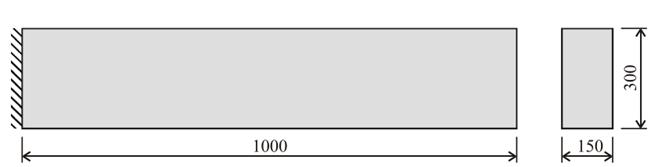Source: The authors.

Figure 1 Viscoelastic cantilever beam geometry.

The numerical simulations ware performed using triangular element (constant strain triangular elements - CST) meshes to discretize the domain of the structures. In order to obtain the suitable refined mesh a convergence analysis was performed until no significant difference was observed for two levels of refinement. Once defined the configuration of the mesh for each tested beam model, this mesh was used for the both formulations - GHM and ADF. The refined mesh has 602,302 physical dof and 1,800,000 dissipative dof, 2,402,302 dof total. A schema of the used mesh is presented in Fig. 2. The viscoelastic triangular elements matrices were obtained with eq. (6), for GHM Model, and eq. (14), for ADF Model.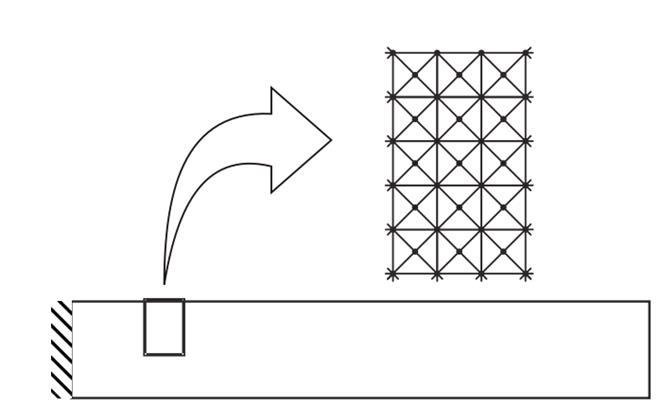Source: The authors.

Figure 2 Structural Finite Element discretization for validation tests.

In order to better evaluate the accuracy of the analyzed methods, two VEM were used for the validation. For GHM’s and ADF’s tests, materials 1 and 2, respectively, were applied. Tables 1 and 2 present the parameters of these materials used in eq. (6) and (13) to obtain the VEM cantilever models. This strategy was adopted due to the differences in the expressions for the complex modulus for the two formulations.

Table 1 Material parameters used in validation tests of GHM model.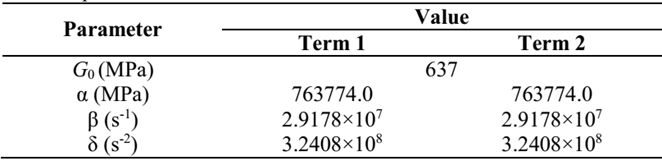Source: The authors.

Table 2 Material parameters used in validation tests of ADF model.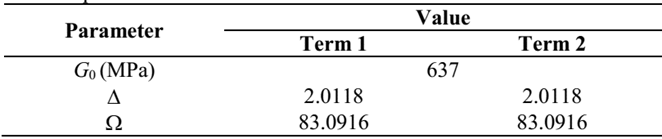Source: The authors.

GHM and ADF formulations allow time domain equations for VEM. However, using their respective frequency domain equations, it is possible to apply classical discrete solutions to compare and evaluate the quality of GHM and ADF results, as is explained below.

Starting from the dynamic equilibrium equations, using the complex excitation one has the classical discrete equation:

where M is the structure mass matrix; is the frequency dependent stiffness matrix on the structure; is the harmonic excitation vector having ω as the excitation frequency and . It can be noticed that, if the mechanical properties of the structure are not frequency dependent, , which is not the case of VEM.

Using GHM or ADF, eq. (14) may be rewritten as:

where, and the mass, stiffness and damping matrices for GHM or ADF are respectively. It is important to notice that the stiffness matrixes in the GHM or ADF formulations are not frequency dependent.

The frequency domain displacements, q, may be obtained by solving eq. (15) and (16), resulting in eq. (17) and (18), respectively:

By solving eq. (16) and (17) for the two beam models, the graphs in Fig. 3 may be achieved. It is possible to observe that, for all tested beam models, ADF and GHM formulations allow identical results when compared to the respective classical responses, supporting the accuracy of applied methods. Fig. 3.a and 3.b were achieved observing the vertical nodal displacements at the free end of the beam models, with only one nodal harmonic transversal load also at the free end. As can be seen in this figure both models produce the same response on the frequency domain as the Classic model.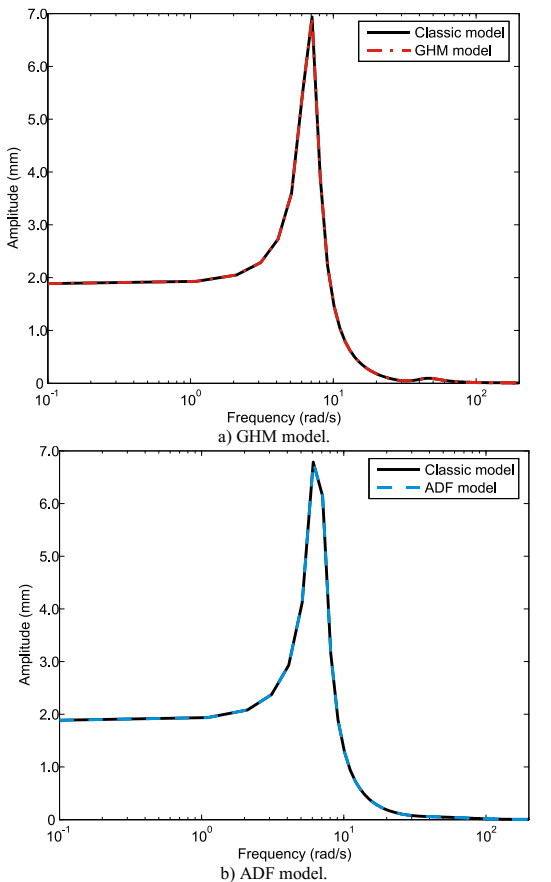Source: The authors.

Figure 3 Density frequency functions for validation tests.

3.2. Time evaluation of numerical methods

Another parameter to compare both methods is the time taken to assemble the models global matrixes. Both methods ware implemented virtually with the same code in MATLAB where each program uses global sparse matrixes; Assembles the global matrixes element-per-element; and for each element, an eigenvalue problem is solved.

In an Intel Core 2 Duo computer with 2.1GHz clock and 2.00Gb RAM the VEM cantilever beam models were evaluated 20 times, running under a Windows Vista operational system, and the total time taken to assemble the global matrixes and impose the boundary conditions were registered. The time taken to solve the time problem and to solve the dynamical system were disregarded.

With these registered times the histograms on Fig. 4 were able to build. For the GHM model the mean time is 8.97h with standard deviation 1.83 and coefficient of variation 0.24; and for the ADF model the mean is 8.00h with the standard deviation 0.79 and coefficient of variation 0.10.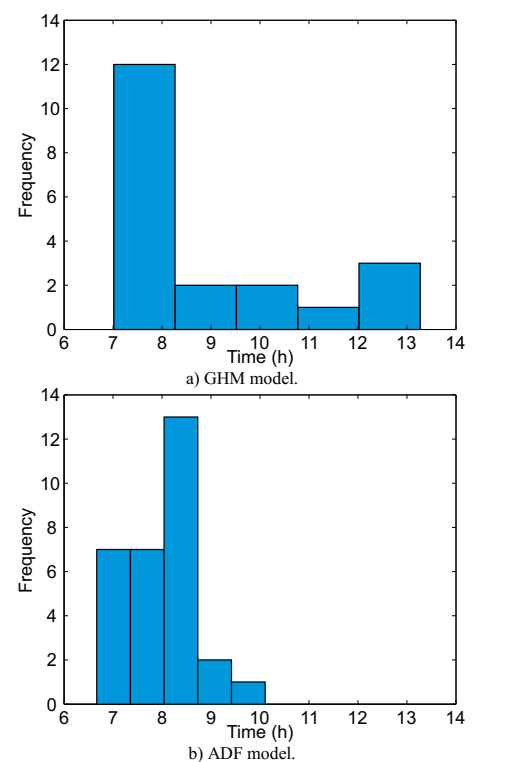Source: The authors.

Figure 4 Time histograms for GHM and ADF viscoelastic cantilever beams.

One can notice that the mean times of both models are close but the ADF model coefficient of variation is about half of the GHM model.

4. Experimental evaluation of VEM models

4.1. Experimental program

In order to evaluate the viscoelastic models, an experimental program was developed. In these laboratory studies, a set of three kinds of sandwich beams was tested. The beams were divided into three groups in accordance with its layer configuration:

a) VS1 beam, with two elastic layers (base beam and clamped restraining layer) and one viscoelastic layer;

b) VS1c beam, with two elastic layers (base beam and free restraining layer) and one viscoelastic layer and;

c) VS2 beam, with three elastic layers (one base beam and two clamped restraining layer).

The layer configurations of each sandwich beam group can be seen at Fig. 5.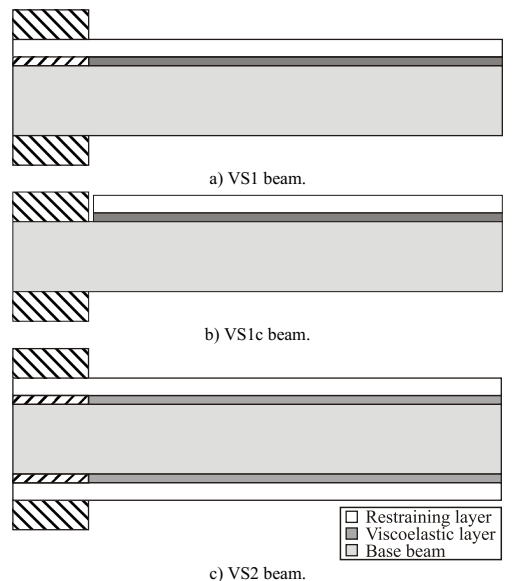Source: The authors.

Figure 5 Longitudinal section of the analyzed beams.

All beams have rectangular cross section and 1140 mm length, working as the elastic base structure with 16,1mm height; viscoelastic layers with 2,0mm height; and elastic constraining layers with 3,17mm height.

The elastic material was aluminum and the viscoelastic material used was VHB 4955 , their mechanical properties are listed in Table 3.

Table 3 Mechanical properties of beam materials.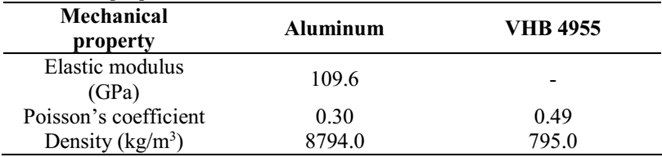Source: The authors.

Two geometrically identical based structures (Base beam A and B) were used for each group of beams. This strategy was applied in order to evaluate the experimental result dispersion. For exact identical Base beams, one should have identical results.

The beams were excited under the action of a hammer impact at 15 cm from cantilever and at the same section the transversal displacements were measured with LVDT sensors, which could register displacements without touching the structure.

For the two base beams (A and B) used as base structures, without damping treatment, the natural frequencies and their damping rates are listed in Table 4, respectively.

Table 4 Natural frequencies and damping ratios of base beams.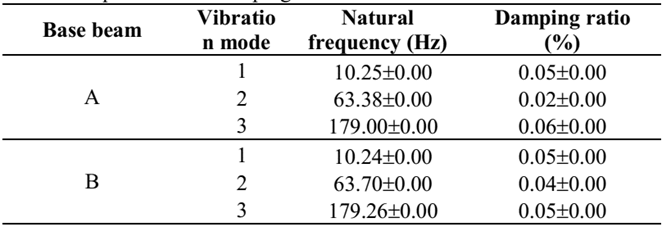Source: The authors.

For the three specimens with damping treatment, their natural frequencies and damping rates are listed in Tables 5-7, respectively.

Table 5 Natural frequencies and damping ratios of VS1 specimens.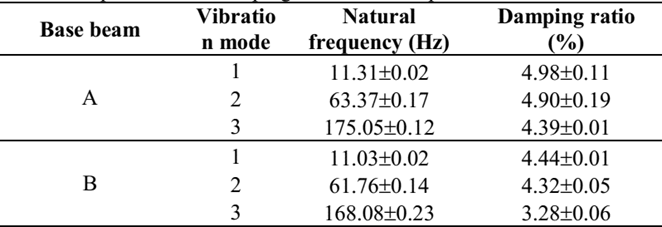Source: The authors.

Table 6 Natural frequencies and damping ratios of VS1c specimens.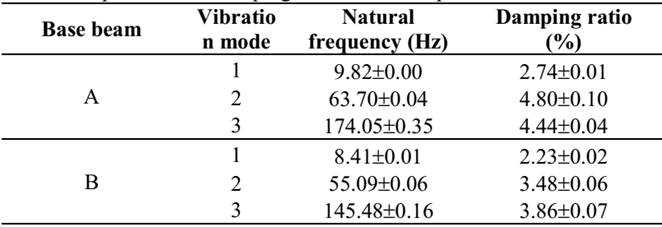Source: The authors.

Table 7 Natural frequencies and damping ratios of VS2 specimens.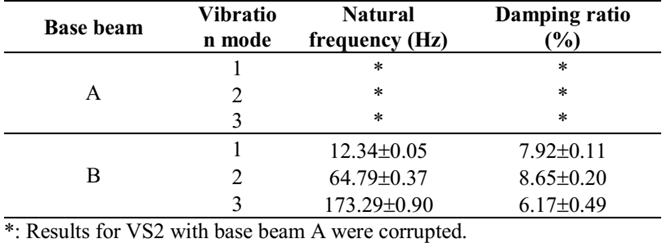Source: The authors.

4.2. Models parameters

There are several methodologies for characterizing the Complex Modulus of viscoelastic materials: ASTM Standard Method , Direct Method  and Indirect Method . These methods basically register, at a given temperature, the temporal responses, when a specimen is submitted into shear or axial deformation .

After the values of Complex Modulus are experimentally determined, one can adjust the curves of the real part of the Complex Modulus and the loss factor for the points obtained experimentally. In the case of the formulation GHM, they are given, in terms of shear modulus, by:

and in the case of the ADF formulation these equations are:

these functions are used to determine the GHM and ADF materials parameters.

In this paper, the Direct Method was applied for frequencies between 0 and 200 Hz. Using data from the experiments, the materials parameters could be determined using the Nonlinear Least Squares Method [19, 20]. These fitted values are shown in Table 8, for the GHM Model, and Table 9, for the ADF Model. Fig. 6 shows two graphics comparing the experimental values and the adjusted curves of G′(ω) and η(ω) for both models.

Table 8 GHM parameters adjusted to the viscoelastic material.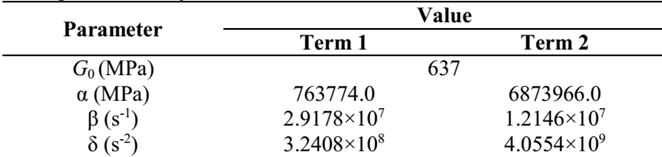Source: The authors.

Table 9 ADF parameters adjusted to the viscoelastic material.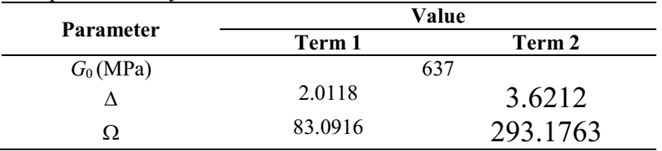Source: The authors.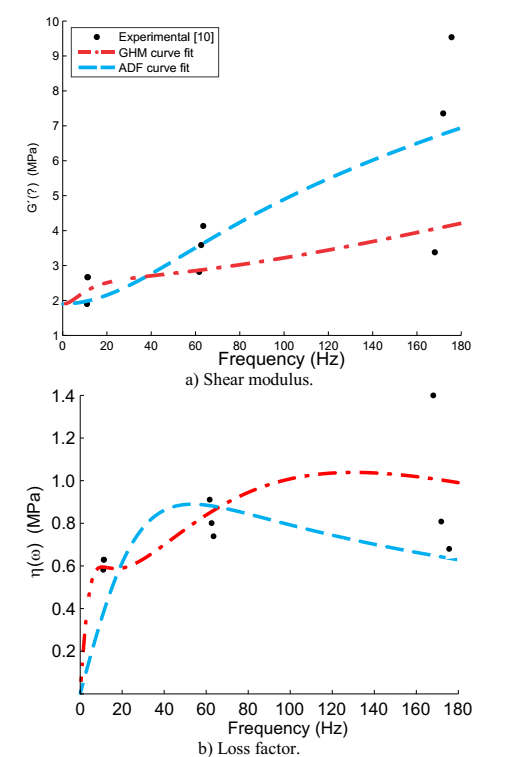Source: The authors

Figure 6 Experimental values and fitted curves of

4.3. Numerical evaluation

The viscoelastic triangular elements matrices were obtained with eq. (6), for GHM Model, and eq. (13), for ADF Model. The discretized beams were excited using an impact model, shown in Fig. 7, at 15 cm from cantilever and, at the same point, the transversal displacement along the time was observed.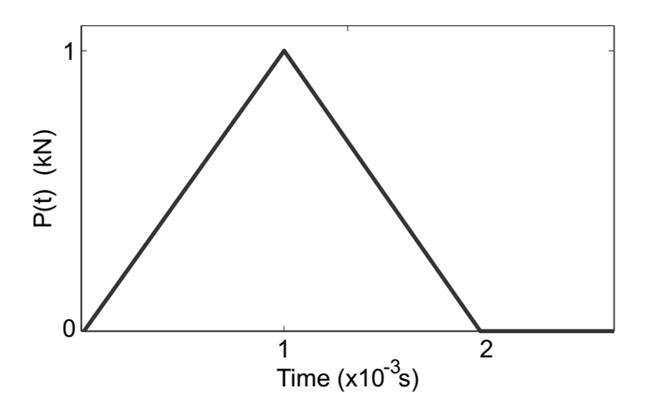Source: The authors.

Figure7 Impact model used to excite the discretized beams.

The same elastic layer mechanical properties and viscoelastic layers ware considered in Table 3 in addition to the GHM and ADF parameters previously listed in this section.

With the models, meshes and mechanical properties described, the time response of beams could be obtained. In order to obtain the natural frequencies and damping rates it was constructed the spectral response; The first three natural frequencies were identified; The time response signal was filtered around these frequencies and; The damping rates were obtained. The relationship between the numerical and experimental data could be seen on Fig. 8 through Fig. 10.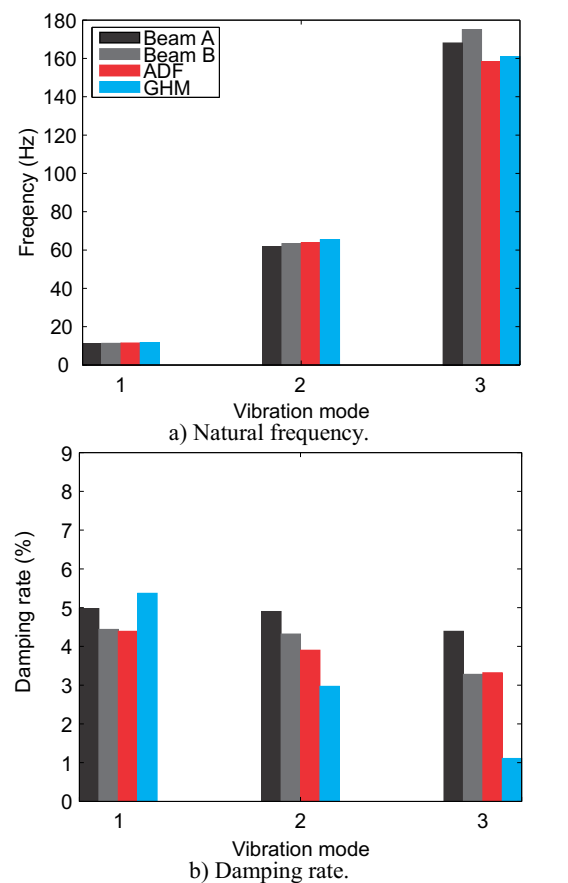Source: The authors.

Figure 8 Relationship between the numerical and experimental data for VS1 beam.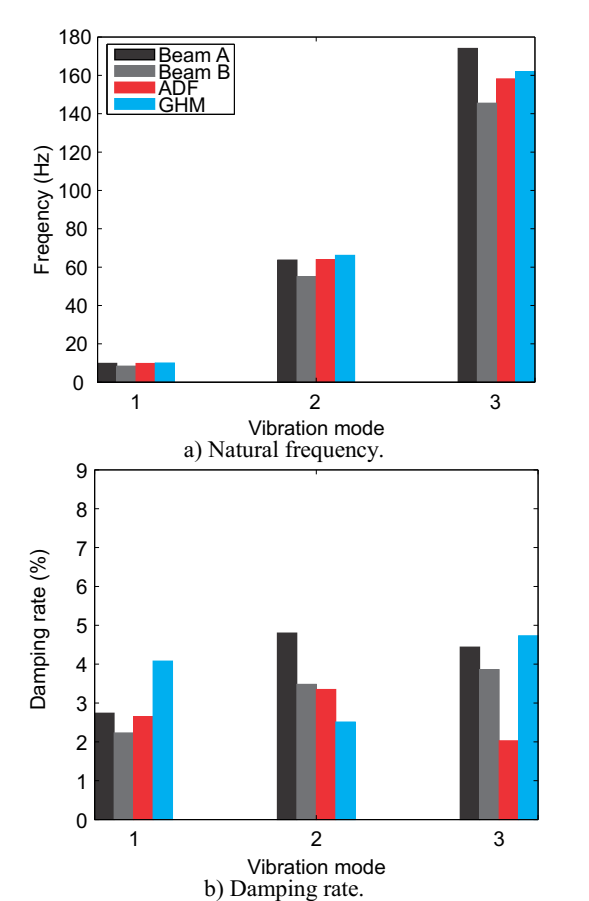Source: The authors.

Figure 9 Relationship between the numerical and experimental data for VS1c beam.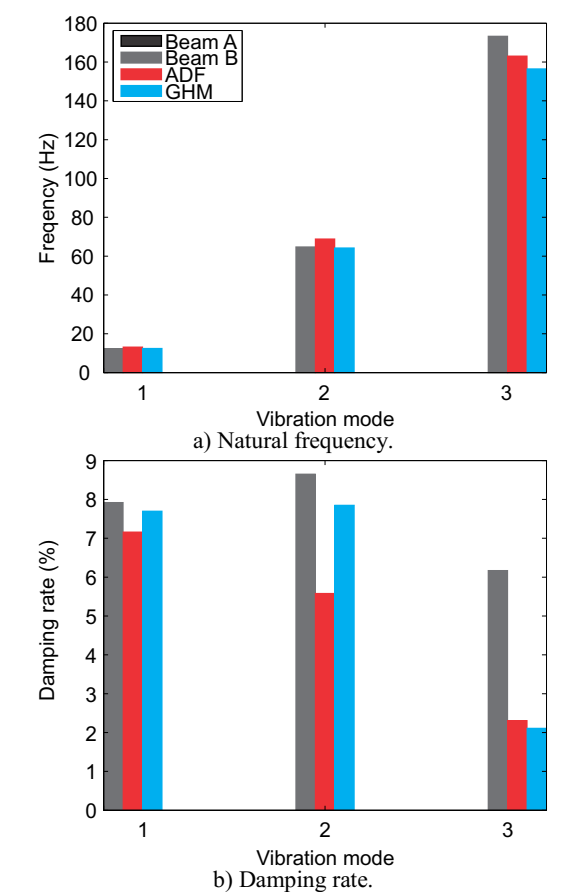Source: The authors.

Figure 10 Relationship between the numerical and experimental data for VS1c beam.

5. Conclusions

This study evaluated the GHM and ADF methods on computational modeling of viscoelastic materials acting as structural vibration dampers. These models were implemented in a finite element code and were observed that both produce the same response on frequency domain and results nearly to those obtained in the experimental program.

Analyzing the obtained responses for the experimentally studied beams, one can observe that natural frequencies obtained with both presented models, in general, have good agreement with the experimental counterpart for the first three modes.

In Table 10, are shown the absolute differences, in terms of damping rates, between the experimental and numerical results. As could be seen, in general, the GHM model presents a better agreement than the ADF model. It could be stated that GHM model evaluated damping rates with differences, in terms of percentual points, between -0,32 and +0,16 for first vibration mode; between -0,80 and -0,71 for second mode and; between -4,06 and -0,52 percentual points for third mode. For the ADF model these differences are between -0,76 and +1,59 for first vibration mode; between -3,07 and -1,64 for second mode and; between -3,86 and +0,55 for third mode.

It is important to emphasize that material functions with two terms in both models were adopted and it is possible to see in Fig. 2 that the curve fit for ADF model was better than that obtained with the GHM model. It can also be highlighted that the Nonlinear Least Squares Method is an iterative curve fit method and a higher number of attempts was needed to get a good fit with the GHM model than with the ADF model.

By analyzing the obtained responses for the cantilever beams, one can observe that despite the good correlation between the fitted curves and the experimental data, the damping factor obtained through the numerical models were, in general, underestimated for both models, while the good agreement of natural frequencies obtained with the models and the experimental values. Obviously these differences cannot be attributed only to curve fitting. Other factors such as: the methodology used on modal identification (in numerical evaluation the exponential decrement methodology was adopted and in laboratory tests the half power method was adopted); 2) Dispersion of experimental results; 3) The Finite Element discretization, also play a significant influence on numerical results.

It could be also observed that the adopted damping treatment, in both cases, considerably increased the damping rates of the structures when compared with the elastic structure without damping treatment. This structural behavior allows the conclusion that viscoelastic materials may be used to reduce vibration oscillations.

Despite the fact that both models provided results close to experimental data and in favor of safety, the curve fit and the results obtained with the ADF model were better than the ones obtained with the GHM model. It seems that considering the formulations presented here the ADF model is more suitable to model viscoelastic materials than the GHM one.

Acknowledgments

Authors would like to thank: CNPq (Conselho Nacional de Desenvolvimento Científico e Tecnológico); UFJF (Federal University of Juiz de Fora); FAPEMIG (Fundação de Amparo à Pesquisa do Estado de Minas Gerais) and CAPES (Coordenação de Aperfeiçoamento de Pessoal de Nível Superior) for financial supports.

References

 Friswell, M.I., Dutt, J.K., Adhikari, S. and Lees, A.W., Time domain analysis of a viscoelastic rotor using internal variable models. International Journal of Mechanical Sciences 52, pp. 1319-1324, 2010. DOI:10.1016/j.ijmecsci.2010.06.007 [ Links ]

 Roy, H., Dutt, J.K. and Datta, P.K., Dynamics of a viscoelastic rotor shaft using augmenting thermodynamic fields - A finite element approach. International Journal of Mechanical Sciences 50, pp. 845-853, 2008. DOI: 10.1016/j.ijmecsci.2007.08.007 [ Links ]

 Wang, G., Veeramani, S. and Wereley, N.M., Analysis of sandwich plates with isotropic face plates and a viscoelastic core. Journal of Vibration and Acoustics 122, pp. 305-312, 2000. DOI: 10.1115/1.1303065 [ Links ]

 Wang, Y. and Inman, D.J., Finite element analysis and experimental study on dynamic properties of a composite beam with viscoelastic damping. Journal of Sound and Vibration 332, pp. 6177-6191, 2013. DOI: 10.1016/j.jsv.2013.06.016 [ Links ]

 Gola, D.F. and Hughes, P.C., Dynamics of viscoelastic structures - a time-domain, finite element formulation. Journal of Applied Mechanics 52, pp. 897-906, 1985. DOI: 10.1115/1.3169166 [ Links ]

 McTavish, D.J. and Hughes, P.C., Modeling of linear viscoelastic space structures. Journal of Vibration and Acoustics, 115, pp. 103-113, 1993. DOI: 10.1115/1.2930302 [ Links ]

 Lesieutre, G.A. and Mingori, D.L., Finite element modeling of frequency-dependent materials properties using Augmented Thermodynamics Fields. Journal of Guidance Control and Dynamics 13, pp. 1040-1050, 1990. DOI: 10.2514/3.20577 [ Links ]

 Lesieutre, G.A., Finite elements for dynamic modeling of uniaxial rods with frequency dependent material properties. International Journal of Solids and Structures 29, pp. 1567-1579, 1992. DOI: 10.1016/0020-7683(92)90134-F [ Links ]

 Lesieutre, G.A. and Bianchini, E., Time domain modeling of linear viscoelasticity using anelastic displacement fields. Journal of Vibration Acoustical 117, pp. 424-430, 1993. DOI: 10.1115/1.2874474 [ Links ]

 Lesieutre, G.A. and Govindswamy, K., Finite element modeling of frequency-dependent and temperature-dependent dynamic behavior of viscoelastic material in simple shear. International Journal of Solids and Structures 33, pp. 419-432, 1996. DOI: 10.1016/0020-7683(95)00048-F [ Links ]

 Lesieutre, G.A. and Lee, U., A finite element for beams having segmented active constrained layers with frequency-dependent viscoelastics. Smart Materials and Structures 5, pp. 615-627, 1996. DOI: 10.1177/1077546029189 [ Links ]

 Biot, M.A., Variational principles in irreversible thermodynamics with application to viscoelasticity. Phisical Review 97, pp. 1463-1469, 1955. DOI: 10.1103/PhysRev.97.1463 [ Links ]

 Barbosa, F.S., Computational modeling of structures with viscoelastic damping layers (in Portuguese). PhD dissertation. Instituto Alberto Luiz Coimbra de Pós-Graduação e Pesquisa de Engenharia, Federal University of Rio de Janeiro (UFRJ), Rio de Janeiro, Brazil, 2002. [ Links ]

 Borges, F., Roitman, N., Magluta, C., Castello, D. and Franciss, R. Reduction of vibration through the use of viscoelastic materials (in Portuguese). Proceedings of XXXII CILAMCE, 2011. [ Links ]

 ASTM. Standard test method for measuring vibration-damping properties of materials. ASTM, 1993. [ Links ]

 Faisca, R.G., Characterization of viscoelastic materials as structural dampers (in Portuguese). MSc. Thesis. Instituto Alberto Luiz Coimbra de Pós-Graduação e Pesquisa de Engenharia, Federal University of Rio de Janeiro (UFRJ), Rio de Janeiro, Brazil, 1998. [ Links ]

 Masterson, P.A. and Miles, R.N., Method for the determination of complex shear modulus of viscoelastic adhesives. Proceedings of 2nd Annual International Symposium on Smart Structures and Materials, Society of Photo-optical Instrumentation Engineers (SPIE), 1995. [ Links ]

 Jones, D.I.G., Handbook of viscoelastic vibration damping. John Wiley and Sons, 2001. [ Links ]

 Coleman, T.F. and Li, Y., On the convergence of reflective Newton methods for large-scale nonlinear minimization subject to bounds. Mathematical Programming, 67, 1994. DOI: 10.1007/BF01582221 [ Links ]

 Coleman, T.F. and Li, Y., An interior, trust region approach for nonlinear minimization subject to bounds. SIAM Journal on Optimization, 6, 1996. DOI: 10.1137/0806023 [ Links ]

How to cite: Felippe, W., Barbosa, F., Magluta, C., Roitman, N. and Borges, F., Numerical comparisons of sandwich viscoelastic beam models.. DYNA, 86(208), pp. 28-36, January - March, 2019

W. Felippe, received the BSc. Eng in Civil Engineering in 2008, the MSc. degree in Computational Modeling in 2012, and the PhD degree in Computational Modeling in 2016, all of them from the Federal University of Juiz de Fora. Juiz de Fora, Brazil. Since 2013, he is a professor in the Civil Engineering Department, University of Barra, Brazil. His research interests include: simulation and modeling of viscoelastic materials; structural dynamics; and optimization using metaheuristics. ORCID: 0000-0002-3499-3499

F. barbosa, received the BSc. Eng in Civil Engineering in 1994 from the Federal University of Juiz de Fora, the MSc. degree and the PhD degree in Civil Engineering in 1996 and 2000, from the Federal University of Rio de Janeiro, Brazil. He worked as postdoctoral researcher between 2001 and 2002 in Laboratoire Central des Ponts et Chaussées (LCPC), France. He is currently professor in the Federal University of Juiz de Fora, Brazil. His research interests include: simulation and modeling of viscoelastic materials; structural dynamics; and signal processing. ORCID: 0000-0002-7991-8425

C. Magluta, received the BSc. Eng in Civil Engineering in 1973, the MSc. degree and the PhD degree in Civil Engineering in 1985 and 1993, from the Federal University of Rio de Janeiro, Brazil. He is currently professor in the Federal University of Rio de Janeiro, Brazil. His research interests include: structural dynamics; experimental analysis of structures; and structural vibration. ORCID: 0000-0002-5184-8648

N. Roitman, received the BSc. Eng in Civil Engineering in 1973 from the Pontifical Catholic University of Rio de Janeiro, Brazil, the MSc. degree and the PhD degree in Civil Engineering in 1979 and 1985, from the Federal University of Rio de Janeiro, Brazil. Since 1999 he is professor in the Federal University of Rio de Janeiro. His research interests include: structural dynamics; experimental analysis of structures; and signal processing. ORCID: 0000-0001-7558-8134

F. Borges, received the BSc. in Math in 2004 from the State University of Rio de Janeiro, the MSc. degree in Civil Engineering in 2010, from the Federal University of Rio de Janeiro, Brazil. Since 2012 she is professor in the Veiga de Almeida University, Brazil. Her research interest include: structural dynamics; experimental analysis of structures; and modeling of viscoelastic materials. ORCID: 0000-0002-6529-5968

Recibido: 04 de Septiembre de 2017; Revisado: 21 de Septiembre de 2018; Aprobado: 25 de Noviembre de 2018This is an open-access article distributed under the terms of the Creative Commons Attribution License